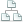Home >Tips >Excel 2016 from Scratch >Bind datasheets ASite map

# An Excel tutorial by Peter KalmstromIn this demo in the Excel 2016 from Scratch series Peter Kalmstrom explains how to apply formulas in different sheets and combine content from cells in two different sheets one formula.

Peter uses IT expenses as an example. He calculates the total of one sheet, fetches the same value into another sheet and also calculates sums for separate rows.

### Exercise

If you want to try the exercise yourself, please download the Excel file Peter uses in the demo.

### Content

This is what Peter shows in the demo below:
• How to use AutoSum for a column.
• How to fetch a value from another sheet to a formula by clicking in the cell.
• How to calculate a sum for separate rows.
• How to hide decimals.
Peter uses Excel 2016 for his demo, but the Excel basics are the same for earlier versions of Excel.